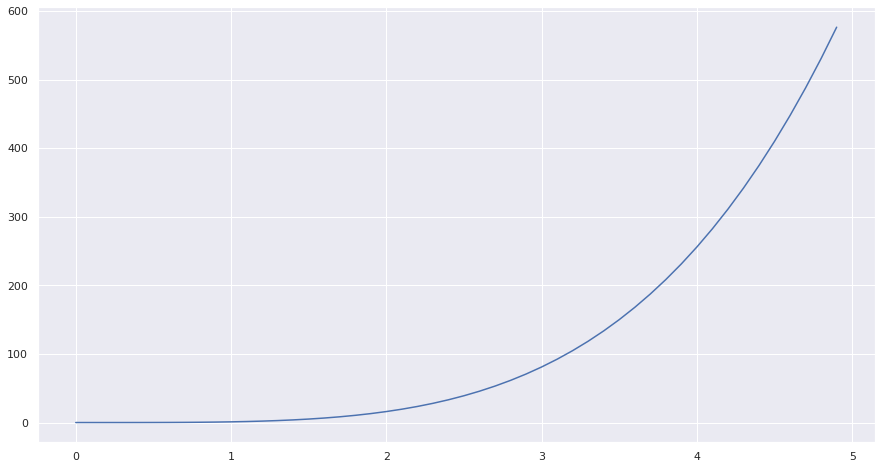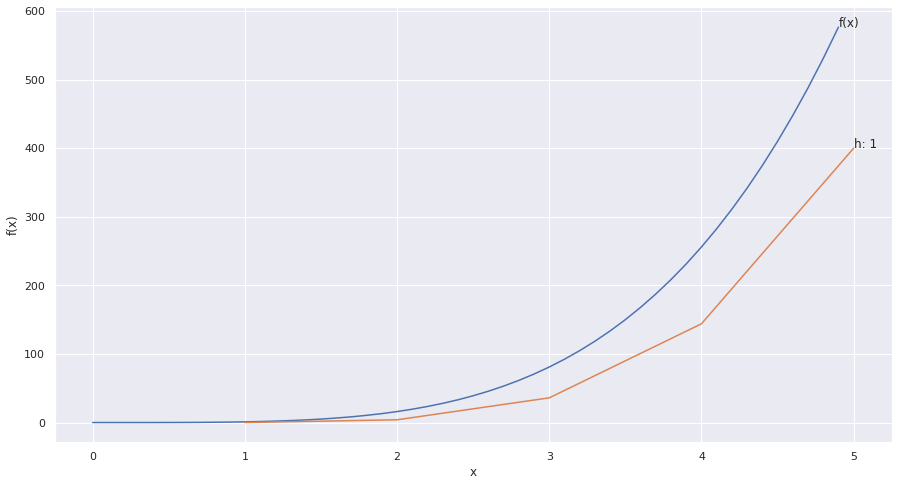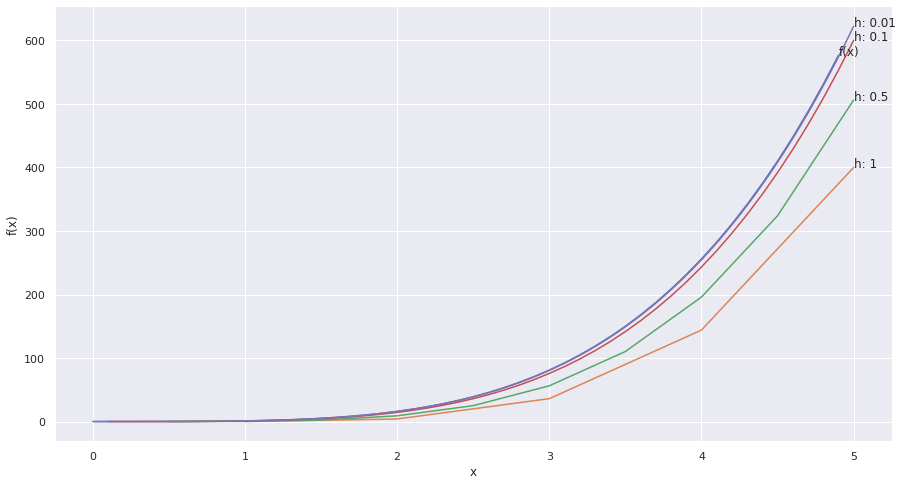Differential equations are fascinating but it’s always hard to get your head around them. Let’s consider the equation

$$y = x^4$$Consider the derivate of this equation:

$$\frac{dy}{dx} = 4x^3$$

So with this we can always know the rate of change of y w.r.t x. For e.g. the rate of change of y w.r.t x at x = 5 would be $\frac{dy}{dx} = 4 * 5^3 = 500$.

Now that was easy, but the question does arise, what if we only knew $\frac{dy}{dx}$ and $y_{0}$, would it be possible to reverse-engineer the graph that we plotted?

So we know that the derivate of y w.r.t x can be written as:

$$\frac{dy}{dx} = \lim_{h \to 0} \frac{f(x + h) - f(x)}{h}$$

This means this holds true for very small values of h. Let’s call $\frac{dy}{dx} = f’(x)$, simplifying the above equation:

$$\frac{f(x + h) - f(x)}{h} = f’(x)$$ $$f(x + h) - f(x) = h f’(x)$$ $$f(x + h) = f(x) + h f’(x)$$

If we denote $f(x + h)$ as $y_{n+1}$, $f(x)$ would be $y_{n}$ our equation would now be:

$$y_{n+1} = y_{n} + h f’(x_{n})$$

This is the base for Euler’s Method, as this lets us extrapolate $y_{n}$ from $y_{0}$ given that we know $\frac{dy}{dx}$.

$$y_{1} = y_{0} + h f’(x_{0})$$ $$y_{2} = y_{1} + h f’(x_{1})$$ $$y_{3} = y_{2} + h f’(x_{2})$$ $$…$$ $$y_{n+1} = y_{n} + h f’(x_{n})$$

Now let’s get back to our example, we know

$$\frac{dy}{dx} = 4 * x^3$$ $$y_{0} = f(x_{0}) = 0$$

using this we can formulate, using a step size (h) of 1

$$y_{1} = 0 + 1 f’(0) = 0 + 1 * (0) = 0$$ $$y_{2} = 0 + 1 f’(1) = 0 + 1 * (4) = 4$$ $$y_{3} = 1 + 1 f’(2) = 4 + 1 * (32) = 36$$ $$…$$ $$y_{n+1} = y_{n} + 1 f’(x_{n})$$

Which will look like this:All we need to do next, is to try the same with different sizes of h. Let’s try for values [1, 0.5, 0.1, 0.01].If you notice, you’ll see that as h gets smaller the approximated function is closer to f(x).

This is the Euler’s method of approximating/solving Ordinary Differential Equations.

Code used to generate the graphs above:

import numpy as np
import matplotlib.pyplot as plt
import seaborn as sns

fx = lambda x: math.pow(x, 4)
dydx = lambda x, y: 4 * math.pow(x, 3)
y0 = 0

x = list(np.arange(0, 5, 0.1))
y = list(map(fx, x))

def euler(fn, y0, h):
y_old = y0
x_old = 0
while True:
y_new = y_old + h * fn(x_old, y_old)
x_old = x_old + h
y_old = y_new
yield(x_old, y_old)

final = 5
h_values = [1, 0.5, 0.1, 0.01]
samples = {}

for h in h_values:
gn = euler(dydx, y0, h)
data = []
for i in range(int(final/h)):
data.append(next(gn))
samples[h] = data

plt.figure(figsize=(15,8))
sns.lineplot(x=x, y=y)
plt.text(x[-1], y[-1], 'f(x)')
for h, data in samples.items():
sns.lineplot(x=[arr for arr in data], y=[arr for arr in data])
plt.text(data[-1], data[-1], 'h: {}'.format(h))
plt.ylabel('f(x)')
plt.xlabel('x')
plt.show()


539 Words

2020-05-02 10:13 +0000